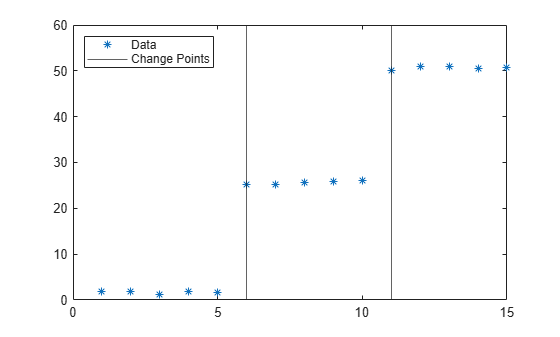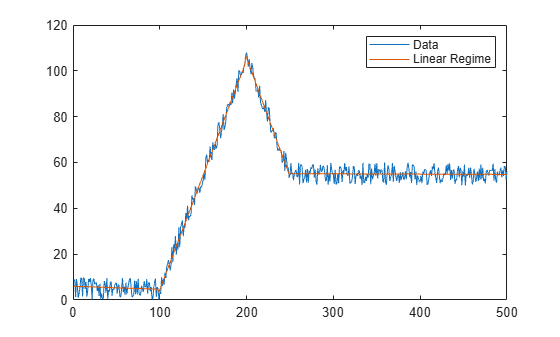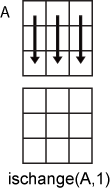# ischange

Find abrupt changes in data

## Syntax

``TF = ischange(A)``
``TF = ischange(A,method)``
``TF = ischange(___,dim)``
``TF = ischange(___,Name,Value)``
``[TF,S1] = ischange(___)``
``[TF,S1,S2] = ischange(___)``

## Description

example

````TF = ischange(A)` returns a logical array whose elements are logical `1` (`true`) when there is an abrupt change in the mean of the corresponding elements of `A`.```

example

````TF = ischange(A,method)` specifies how to define a change point in the data. For example, `ischange(A,'variance')` finds abrupt changes in the variance of the elements of `A`.```

example

````TF = ischange(___,dim)` specifies the dimension of `A` to operate along for either of the previous syntaxes. For example, `ischange(A,2)` computes change points for each row of a matrix `A`.```

example

````TF = ischange(___,Name,Value)` specifies additional parameters for finding change points using one or more name-value arguments. For example, `ischange(A,'MaxNumChanges',m)` detects no more than `m` change points.```

example

````[TF,S1] = ischange(___)` also returns information about the line segments in between change points. For example, `[TF,S1] = ischange(A)` returns a vector `S1` containing the mean of data between change points of a vector `A`.```

example

````[TF,S1,S2] = ischange(___)` returns additional information about the line segments in between change points. For example, `[TF,S1,S2] = ischange(A)` returns a vector `S1` that contains the mean for each segment, as well as a vector `S2` that contains the variance for each segment of a vector `A`.```

## Examples

collapse all

Create a vector of noisy data, and compute the abrupt changes in the mean of the data.

```A = [ones(1,5) 25*ones(1,5) 50*ones(1,5)] + rand(1,15); TF = ischange(A)```
```TF = 1x15 logical array 0 0 0 0 0 1 0 0 0 0 1 0 0 0 0 ```

To compute the mean of the data in between change points, specify a second output argument.

```[TF,S1] = ischange(A); plot(A,'*') hold on stairs(S1) legend('Data','Segment Mean','Location','NW')```Create a vector of noisy data, and compute abrupt changes in the slope and intercept of the data. Setting a large detection threshold reduces the number of change points detected due to noise.

```A = [zeros(1,100) 1:100 99:-1:50 50*ones(1,250)] + 10*rand(1,500); [TF,S1,S2] = ischange(A,'linear','Threshold',200); segline = S1.*(1:500) + S2; plot(1:500,A,1:500,segline) legend('Data','Linear Regime')```As an alternative to providing a threshold value, you also can specify the maximum number of change points to detect.

`[TF,S1,S2] = ischange(A,'linear','MaxNumChanges',3);`

Compute abrupt changes in the mean for each row of a matrix.

`A = diag(25*ones(5,1)) + rand(5,5)`
```A = 5×5 25.8147 0.0975 0.1576 0.1419 0.6557 0.9058 25.2785 0.9706 0.4218 0.0357 0.1270 0.5469 25.9572 0.9157 0.8491 0.9134 0.9575 0.4854 25.7922 0.9340 0.6324 0.9649 0.8003 0.9595 25.6787 ```
`TF = ischange(A,2)`
```TF = 5x5 logical array 0 1 0 0 0 0 1 1 0 0 0 0 1 1 0 0 0 0 1 1 0 0 0 0 1 ```

## Input Arguments

collapse all

Input data, specified as a vector, matrix, multidimensional array, table, or timetable.

Data Types: `single` | `double` | `table` | `timetable`

Change detection method, specified as one of these values:

• `'mean'` — Find abrupt changes in the mean of the data.

• `'variance'` — Find abrupt changes in the variance of the data.

• `'linear'` — Find abrupt changes in the slope and intercept of the data.

Operating dimension, specified as a positive integer scalar. If no value is specified, then the default is the first array dimension whose size does not equal 1.

Consider an `m`-by-`n` input matrix, `A`:

• `ischange(A,1)` detects change points based on the data in each column of `A` and returns an `m`-by-`n` matrix.• `ischange(A,2)` detects change points based on the data in each row of `A` and returns an `m`-by-`n` matrix.For table or timetable input data, `dim` is not supported and operation is along each table or timetable variable separately.

### Name-Value Arguments

Specify optional pairs of arguments as `Name1=Value1,...,NameN=ValueN`, where `Name` is the argument name and `Value` is the corresponding value. Name-value arguments must appear after other arguments, but the order of the pairs does not matter.

Before R2021a, use commas to separate each name and value, and enclose `Name` in quotes.

Example: `TF = ischange(A,'MaxNumChanges',5)`

Data Options

collapse all

Sample points, specified as a vector of sample point values or one of the options in the following table when the input data is a table. The sample points represent the x-axis locations of the data, and must be sorted and contain unique elements. Sample points do not need to be uniformly sampled. The vector ```[1 2 3 ...]``` is the default.

When the input data is a table, you can specify the sample points as a table variable using one of these options:

Indexing SchemeExamples

Variable name:

• A string scalar or character vector

• `"A"` or `'A'` — A variable named `A`

Variable index:

• An index number that refers to the location of a variable in the table

• A logical vector. Typically, this vector is the same length as the number of variables, but you can omit trailing `0` or `false` values

• `3` — The third variable from the table

• `[false false true]` — The third variable

Function handle:

• A function handle that takes a table variable as input and returns a logical scalar

• `@isnumeric` — One variable containing numeric values

Variable type:

• `vartype("numeric")` — One variable containing numeric values

Note

This name-value argument is not supported when the input data is a `timetable`. Timetables use the vector of row times as the sample points. To use different sample points, you must edit the timetable so that the row times contain the desired sample points.

Example: ```ischange([1 2 3 4 5 6],'linear','SamplePoints',[1 2 3 10 20 30])```

Example: `ischange(T,'linear','SamplePoints',"Var1")`

Data Types: `single` | `double` | `datetime` | `duration`

Table variables to operate on, specified as one of the options in this table. The `DataVariables` value indicates which variables of the input table to examine for change points. The data type associated with the indicated variables must be `double` or `single`.

The first output `TF` contains `false` for variables not specified by `DataVariables` unless the value of `OutputFormat` is `'tabular'`.

Indexing SchemeExamples

Variable names:

• A string, character vector, or cell array

• A `pattern` object

• `"A"` or `'A'` — A variable named `A`

• `["A","B"]` or `{'A','B'}` — Two variables named `A` and `B`

• `"Var"+digitsPattern(1)` — Variables named `"Var"` followed by a single digit

Variable index:

• An index number that refers to the location of a variable in the table

• A vector of numbers

• A logical vector. Typically, this vector is the same length as the number of variables, but you can omit trailing `0` or `false` values

• `3` — The third variable from the table

• `[2 3]` — The second and third variables from the table

• `[false false true]` — The third variable

Function handle:

• A function handle that takes a table variable as input and returns a logical scalar

• `@isnumeric` — All the variables containing numeric values

Variable type:

• `vartype("numeric")` — All the variables containing numeric values

Example: ```ischange(T,'DataVariables',["Var1" "Var2" "Var4"])```

Output data type, specified as one of these values:

• `'logical'` — For table or timetable input data, return the output `TF` as a logical array.

• `'tabular'` — For table input data, return the output `TF` as a table. For timetable input data, return the output `TF` as a timetable.

For vector, matrix, or multidimensional array input data, `OutputFormat` is not supported.

Example: `ischange(T,'OutputFormat','tabular')`

Change Point Options

collapse all

Change point threshold, specified as a nonnegative scalar. Increasing the threshold greater than 1 produces fewer change points.

The threshold value determines the number of detected change points and cannot be specified when `MaxNumChanges` is specified.

Maximum number of change points to detect, specified as a positive integer scalar. `ischange` uses an automatic threshold that computes no more than the specified value of change points, thus `Threshold` cannot be specified when `MaxNumChanges` is specified.

## Output Arguments

collapse all

Change point indicator, returned as a vector, matrix, multidimensional array, table, or timetable.

`TF` is the same size as `A` unless the value of `OutputFormat` is `'tabular'`. If the value of `OutputFormat` is `'tabular'`, then `TF` only has variables corresponding to the `DataVariables` specified.

Data Types: `logical`

Mean or slope of data between change points, returned as a vector, matrix, multidimensional array, table, or timetable.

• If the change point detection method is `'mean'` or `'variance'`, then `S1` contains the mean for each segment.

• If the method is `'linear'`, then `S1` contains the slope for each segment.

`S1` has the same type as the input data.

Data Types: `double` | `single` | `table` | `timetable`

Variance or intercept of data between change points, returned as a vector, matrix, multidimensional array, table, or timetable.

• If the change point detection method is `'mean'` or `'variance'`, then `S2` contains the variance for each segment.

• If the method is `'linear'`, then `S2` contains the intercept for each segment.

`S2` has the same type as the input data.

Data Types: `double` | `single` | `table` | `timetable`

collapse all

### Change Points

A vector of data A contains a change point if it can be split into two segments A1 and A2 such that

`$C\left({A}_{1}\right)+C\left({A}_{2}\right)+\tau `

$\tau$ is the threshold value specified by the `Threshold` parameter, and C represents a cost function.

For example, the cost function for detecting abrupt changes in the mean is $C\left(x\right)=N\mathrm{var}\left(x\right)$, where N is the number of elements in a vector x. The cost function measures how well a segment is approximated by its mean.

`ischange` iteratively minimizes the sum of the cost functions to determine the number of change points k and their locations such that

`$C\left({A}_{1}\right)+C\left({A}_{2}\right)+...+C\left({A}_{k}\right)+k\tau `

 Killick R., P. Fearnhead, and I.A. Eckley. "Optimal detection of changepoints with a linear computational cost." Journal of the American Statistical Association. Vol. 107, Number 500, 2012, pp.1590-1598.